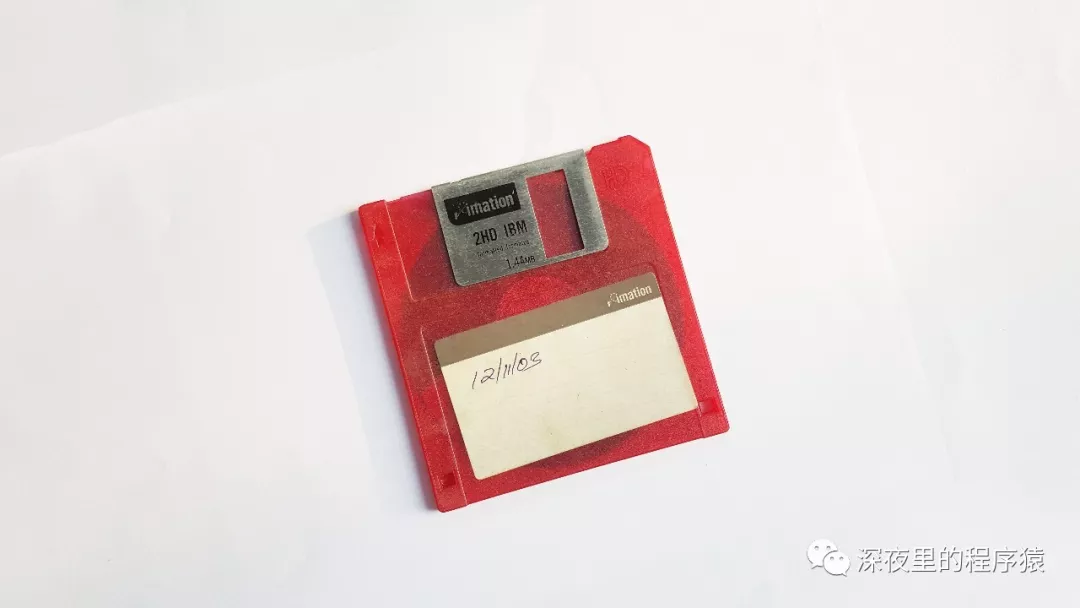``//创建对象``ThreadLocal threadLocal = new ThreadLocal<String>();``//设值``threadLocal.set("深夜里的程序猿");``//取值``threadLocal.get(); // get  深夜里的程序猿``

``public void set(T value) {``        Thread t = Thread.currentThread();``        ThreadLocalMap map = getMap(t);``        if (map != null)``            map.set(this, value);``        else``            createMap(t, value);``    }``

``public T get() {``        Thread t = Thread.currentThread();``        ThreadLocalMap map = getMap(t);``        if (map != null) {``            ThreadLocalMap.Entry e = map.getEntry(this);``            if (e != null) {``                @SuppressWarnings("unchecked")``                T result = (T)e.value;``                return result;``            }``        }``        return setInitialValue();``    }``

​​​​​​​

``ThreadLocalMap getMap(Thread t) {``        return t.threadLocals;``}``

````static class Entry extends WeakReference<ThreadLocal<?>> {``        /** The value associated with this ThreadLocal. */``        Object value;`
`        Entry(ThreadLocal<?> k, Object v) {``            super(k);``            value = v;``        }`` }````

``private void remove(ThreadLocal<?> key) {``      Entry[] tab = table;``      int len = tab.length;``      int i = key.threadLocalHashCode & (len-1);``      for (Entry e = tab[i];``           e != null;``           e = tab[i = nextIndex(i, len)]) {``          if (e.get() == key) {``              //清理对ThreadLocal的弱引用``              e.clear();``              //清理key为null的元素``              expungeStaleEntry(i);``              return;``          }``      }``  }``